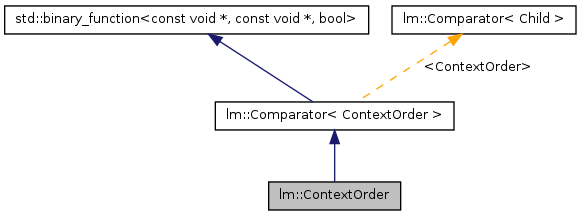# lm::ContextOrder Class Reference

`#include <compare.hh>`

Inheritance diagram for lm::ContextOrder:[legend]
Collaboration diagram for lm::ContextOrder:[legend]

List of all members.

## Public Member Functions

ContextOrder (std::size_t order)
bool Compare (const WordIndex *lhs, const WordIndex *rhs) const

## Detailed Description

N-gram comparator that compares n-grams according to the reverse (suffix) order of the n-gram context.

This comparator compares n-grams lexicographically, one word at a time, beginning with the penultimate word of each n-gram and ending with the first word of each n-gram; finally, this comparator compares the last word of each n-gram.

Some examples of n-gram comparisons as defined by this comparator:

• a b c == a b c
• a b c < a b d
• a b c < a d b
• a b c > a b b
• a b c > x a c
• a b c < x y z

Definition at line 103 of file compare.hh.

## Constructor & Destructor Documentation

 lm::ContextOrder::ContextOrder ( std::size_t order ) ` [inline, explicit]`

Constructs a comparator capable of comparing two n-grams.

Parameters:
 order Number of words in each n-gram

Definition at line 111 of file compare.hh.

## Member Function Documentation

 bool lm::ContextOrder::Compare ( const WordIndex * lhs, const WordIndex * rhs ) const` [inline]`

Compares two n-grams lexicographically, one word at a time, beginning with the penultimate word of each n-gram and ending with the first word of each n-gram; finally, this comparator compares the last word of each n-gram.

Parameters:
 lhs A pointer to the n-gram on the left-hand side of the comparison rhs A pointer to the n-gram on the right-hand side of the comparison

Definition at line 121 of file compare.hh.

References lm::Comparator< ContextOrder >::order_.

The documentation for this class was generated from the following file:
• /disk4/html/www/moses/doxygen/mosesdecoder/lm/common/compare.hh

Generated on Thu Jul 6 00:32:39 2017 for Moses by1.5.9